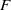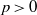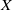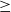Hostname: page-component-7d684dbfc8-4nnqn Total loading time: 0 Render date: 2023-10-01T15:00:10.473Z Has data issue: false Feature Flags: { "corePageComponentGetUserInfoFromSharedSession": true, "coreDisableEcommerce": false, "coreDisableSocialShare": false, "coreDisableEcommerceForArticlePurchase": false, "coreDisableEcommerceForBookPurchase": false, "coreDisableEcommerceForElementPurchase": false, "coreUseNewShare": true, "useRatesEcommerce": true } hasContentIssue false

# Depth of$F$-singularities and base change of relative canonical sheaves

Published online by Cambridge University Press:  05 March 2013

## Abstract

For a characteristic-$p\gt 0$ variety$X$ with controlled$F$-singularities, we state conditions which imply that a divisorial sheaf is Cohen–Macaulay or at least has depth$\geq$3 at certain points. This mirrors results of Kollár for varieties in characteristic 0. As an application, we show that relative canonical sheaves are compatible with arbitrary base change for certain families with sharply$F$-pure fibers.

Type
Research Article
Information

## Access options

Get access to the full version of this content by using one of the access options below. (Log in options will check for institutional or personal access. Content may require purchase if you do not have access.)

## References

Aberbach, I. M. and Enescu, F., The structure of$F$-pure rings, Math. Z. 250 (4) (2005), 791806, MR2180375.CrossRefGoogle Scholar
Alexeev, V., Limits of stable pairs, Pure Appl. Math. Q. 4 (3)(2008), Special Issue: In honor of Fedor Bogomolov. Part 2, 767–783, 2435844 (2009j:14020).CrossRefGoogle Scholar
Alexeev, V. and Hacon, C., Non-rational centers of log canonical singularities (arXiv:1109.4164).Google Scholar
Bhatt, B., Ho, W., Patakfalvi, Zs and Schnell, C., Moduli of products of stable varieties (arXiv:1206.0438).Google Scholar
Blickle, M., Test ideals via algebras of${p}^{- e}$-liner maps, J. Algebraic Geom. 22 (2013), 4983.CrossRefGoogle Scholar
Blickle, M., Schwede, K. and Tucker, K.,$F$-signature of pairs and the asymptotic behavior of Frobenius splittings (arXiv:1107.1082).Google Scholar
Brion, M. and Kumar, S., Frobenius splitting methods in geometry and representation theory, Progress in Mathematics, Volume 231 (Birkhäuser Boston Inc., Boston, MA, 2005), MR2107324 (2005k:14104).Google Scholar
Bruns, W. and Herzog, J., Cohen–Macaulay rings, Cambridge Studies in Advanced Mathematics, Volume 39 (Cambridge University Press, Cambridge, 1993), MR1251956 (95h:13020).Google Scholar
Conrad, B., Grothendieck duality and base change, Lecture Notes in Mathematics, Volume 1750 (Springer-Verlag, Berlin, 2000), MR1804902 (2002d:14025).CrossRefGoogle Scholar
de Jong, A. J., Smoothness, semi-stability and alterations, Publ. Math. Inst. Hautes Études Sci. (83) (1996), 5193, 1423020 (98e:14011).CrossRefGoogle Scholar
Enescu, F. and Hochster, M., The Frobenius structure of local cohomology, Algebra Number Theory 2 (7) (2008), 721754, MR2460693 (2009i:13009).CrossRefGoogle Scholar
Fedder, R.,$F$-purity and rational singularity, Trans. Amer. Math. Soc. 278 (2) (1983), 461480, MR701505 (84h:13031).Google Scholar
Fujino, O., Introduction to the log minimal model program for log canonical pairs (arXiv:0907.1506).Google Scholar
Gabber, O., Notes on some$t$-structures, in Geometric aspects of Dwork theory. Volume I, II, pp. 711734 (Walter de Gruyter GmbH & Co. KG, Berlin, 2004).Google Scholar
Grothendieck, A., Éléments de géométrie algébrique. IV. Étude locale des schémas et des morphismes de schémas. II, Publ. Math. Inst. Hautes Études Sci. (24) (1965), 231, 0199181 (33 #7330).Google Scholar
Hacon, C. D. and Kovács, S. J., Classification of higher dimensional algebraic varieties, Oberwolfach Seminars, Volume 41 (Birkhäuser Verlag, Basel, 2010), 2675555 (2011f:14025).CrossRefGoogle Scholar
Hara, N. and Watanabe, K.-I., F-regular and F-pure rings vs. log terminal and log canonical singularities, J. Algebraic Geom. 11 (2) (2002), 363392, MR1874118 (2002k:13009).CrossRefGoogle Scholar
Hartshorne, R., Residues and duality, in Lecture notes of a seminar on the work of A. Grothendieck, given at Harvard 1963/64. With an appendix by P. Deligne, Lecture Notes in Mathematics, Volume 20 (Springer-Verlag, Berlin, 1966), MR0222093 (36 #5145).Google Scholar
Hartshorne, R., Generalized divisors on Gorenstein schemes, in Proceedings of Conference on Algebraic Geometry and Ring Theory in honor of Michael Artin, Part III (Antwerp, 1992), Volume 8, pp. 287339 (1994), MR1291023 (95k:14008).Google Scholar
Hassett, B. and Kovács, S. J., Reflexive pull-backs and base extension, J. Algebraic Geom. 13 (2) (2004), 233247, MR2047697 (2005b:14028).CrossRefGoogle Scholar
Hochster, M. and Roberts, J. L., The purity of the Frobenius and local cohomology, Adv. Math. 21 (2) (1976), 117172, MR0417172 (54 #5230).CrossRefGoogle Scholar
Kollár, J., Subadditivity of the Kodaira dimension: fibers of general type, in Algebraic geometry, Sendai, 1985, Adv. Stud. Pure Math., Volume 10, pp. 361398 (North-Holland, Amsterdam, 1987), MR946244 (89i:14029).Google Scholar
Kollár, J., Projectivity of complete moduli, J. Differential Geom. 32 (1) (1990), 235268, 1064874 (92e:14008).CrossRefGoogle Scholar
Kollár, J., Hulls and husks (arXiv:0805.0576).Google Scholar
Kollár, J., A local version of the Kawamata–Viehweg vanishing theorem, Pure Appl. Math. Q. 7 (4)(2011), Special Issue: In memory of Eckart Viehweg, 1477–1494. 2918170.CrossRefGoogle Scholar
Kollár, J., and 14 coauthors, Flips and abundance for algebraic threefolds, Société Mathématique de France, Paris, 1992, Papers from the Second Summer Seminar on Algebraic Geometry held at the University of Utah, Salt Lake City, Utah, August 1991, Astérisque No. 211 (1992), MR1225842 (94f:14013).Google Scholar
Kollár, J. and Kovács, S. J., Log canonical singularities are Du Bois, J. Amer. Math. Soc. 23 (3) (2010), 791813, 2629988.CrossRefGoogle Scholar
Kollár, J. and Mori, S., Birational geometry of algebraic varieties, Cambridge Tracts in Mathematics, Volume 134 (Cambridge University Press, Cambridge, 1998), With the collaboration of C. H. Clemens and A. Corti, Translated from the 1998 Japanese original, MR1658959 (2000b:14018).CrossRefGoogle Scholar
Kovács, S., Irrational centers, Pure Appl. Math. Q. 7 (4)(2011), Special Issue: In memory of Eckart Viehweg, 1495–1515. 2918171.CrossRefGoogle Scholar
Kunz, E., On Noetherian rings of characteristic$p$, Amer. J. Math. 98 (4) (1976), 9991013, MR0432625 (55 #5612).CrossRefGoogle Scholar
Miller, L. E. and Schwede, K., Semi-log canonical vs$F$-pure singularities, J. Algebra 349 (2012), 150164, 2853631.CrossRefGoogle Scholar
Patakfalvi, Zs, Base change behavior of the relative canonical sheaf related to higher dimensional moduli, Algebra Number Theory, in press (arXiv:1005.5207).Google Scholar
Raynaud, M., Contre-exemple au vanishing theorem en caractéristique$p\gt 0$, in C. P. Ramanujam—a tribute, Tata Inst. Fund. Res. Studies in Math., Volume 8, pp. 273278 (Springer, Berlin, 1978), 541027 (81b:14011).Google Scholar
Schwede, K.,$F$-adjunction, Algebra Number Theory 3 (8) (2009), 907950, 2587408 (2011b:14006).CrossRefGoogle Scholar
Schwede, K., Centers of$F$-purity, Math. Z. 265 (3) (2010), 687714, 2644316 (2011e:13011).CrossRefGoogle Scholar
Schwede, K., Test ideals in non-$\mathbb{Q}$-Gorenstein rings, Trans. Amer. Math. Soc. 363 (11) (2011), 59255941.CrossRefGoogle Scholar
Schwede, K. and Smith, K. E., Globally$F$-regular and log Fano varieties, Adv. Math. 224 (3) (2010), 863894, 2628797 (2011e:14076).CrossRefGoogle Scholar
Takagi, S., A characteristic$p$ analogue of plt singularities and adjoint ideals, Math. Z. 259 (2) (2008), 321341, MR2390084 (2009b:13004).CrossRefGoogle Scholar